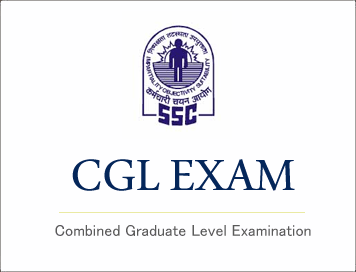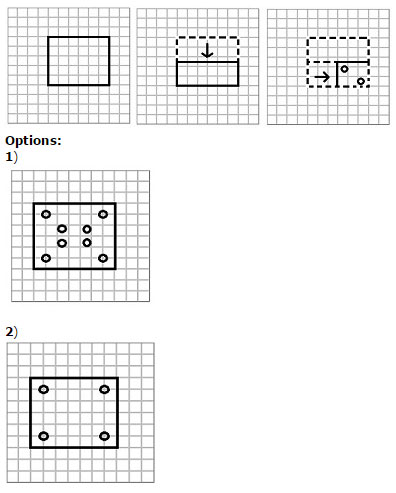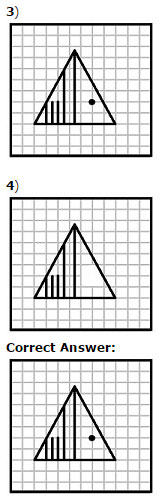# SSC CGL (Tier - 1) Online Exam Paper - 2016 "held on 09 September 2016" Morning Shift (Reasoning)## SSC CGL (Tier - 1) Online Exam Paper - 2016 "held on 09 September 2016" Morning Shift (Reasoning)

EXAM DATE : 9-September-2016
EXAM START TIME :
10:00:00
EXAM NAME :
SSC Examination 2016

Question 1.Select the related word/letters/numbers from the given alternatives:
Magazine : Editor :: Drama : ?

Options:

1) Director
2) Hero
3) Heroine
4) Painter

Question 2.Select the related word/letters/numbers from the given alternatives:
ACEG : IKMO :: QSUW : ?

Options:

1) YZCE
2) YACD
3) YACE
4) YBCE

Question 3.Select the related word/letters/numbers from the given alternatives:
12 : 39 :: 15 : ?

Options:

1) 48
2
) 52
3) 39
4) 51

Question 4.
For the following questions
Find the odd word/letters/number pair from the given alternatives.

Options:

1) Torch
2) Battery
3) Candle
4) Lamp

Question 5.
For the following questions
Find the odd word/letters/number pair from the given alternatives.'

Options:

1) CA
2) FD
3) KI
4) TQ

Question 6.
For the following questions
Find the odd word/letters/number pair from the given alternatives.

Options:

1) 73-61
2) 57-69
3) 47-59
4) 42-29

Question 7.Arrange the following words as per order in the dictionary.
1. Scarf 2.Scene 3.Shell 4.Survey 5.Stream

Options:

1) 1, 2, 4, 5, 3
2) 2, 4, 5, 1, 3
3) 3, 1, 2, 5, 4
4) 1, 2, 3, 5, 4

Correct Answer: 1, 2, 3, 5, 4

Question 8.A series is given, with one term missing. Choose the correct alternative from the given ones that will complete the series.
DCB, HGF, ? , PON

Options:

1) LKJ
2) QRO
3) SUM
4) XZY

## Study Kit for SSC CGL EXAM

Question 9.A series is given, with one term missing. Choose the correct alternative from the given ones that will complete the series.
4, 9, 16, 25, 36, ?

Options:

1) 49
2) 56
3) 21
4) 94

Question 10.A is D's brother. D is B's father. B&C are sisters. How is C related to A?

Options:

1) Cousin
2) Niece
3) Aunt
4) Nephew

Question 11.At a college party 5 girls are sitting in a row. P is to the left of M and to the right of O. R is sitting to the right of N, but to the left of O. Who is sitting in the middle?

Options:

1) O
2) R
3) P
4) M

Question 12.From the given alternative words, select the word which cannot be formed using the letters of the given word:
CONSULTATION

Options:

1) CONSTANT
2) NATION
3) SALUTE
4) STATION

Question 13.If S = 19, SUN = 54 and CAKE = 20 , then MISTAKE = ?

Options:

1) 78
2) 68
3) 59
4) 48

Question 14.If '+' stands for multiplication, '-' stands for addition, 'x' stands for division, then what is the value of 128 + 9 - 16 x 4 = ?

Options:

1) 73
2) 256
3) 1156
4) 1352

Question 15.In this question, some equations are solved on the basis of a certain system. On the same basis find out the correct answer from amongst the four alternatives for the unsolved equation.
6 x 2 x 9 = 269
8 x 7 x 1 = 781
4 x 1 x 3 = ?

Options:

1) 431
2
) 413
3) 341
4) 143

Question 16.Select the missing numbers from the given alternativesOptions:

1) 143
2) 169
3) 455
4) 545

Question 17.To attend an exam, Sudhir reached the school by travelling 5 km towards South, and after a sharp left turn, he travelled for about 10 km. He again made a sharp left turn and reached in front of the school by travelling 5 km more. Which direction is Sudhir's starting point from the school?

Options:

1) East
2) West
3) North
4) South

Question 18.Consider the given statement/s to be true and decide which of the given conclusions/assumptions can definitely be drawn from the given statement.
Statements: 1. All books are novels.
2. Some novels are poems.
Conclusions: I. Some books are poems.
II. Some poems are novels.

Options:

1) Only conclusion I follows
2) Only conclusion II follows
3) Neither conclusion I nor conclusion II follows
4) Both conclusion I and conclusion II follow

Correct Answer: Only conclusion II follows

Question 19.Which symbol will appear on the face opposite to the face of a circle O in the cube given below?Question 20.Which one of the following diagrams represents the relationship between Insects, Flies and Dogs?Question 21.Which answer figure will complete the pattern in the question figure?Question 22.From the given answer figures, select the one in which the question figure is hidden.Question 23.A piece of paper is folded and cut as shown below in the question figures. From the given answer figures, indicate how it will appear when opened.Question 24.Which of the answer figure is exactly the mirror image of the given figure, when the mirror is held on the line AB ?Question 25.In the question, a word is represented by only one set of numbers as given in any one of the alternatives. The sets of numbers given in the alternatives are represented by two classes of alphabets as in two matrices given below. The columns and rows of Matrix I are numbered from 0 to 4 and that of Matrix II are numbered from 5 to 9. A letter from these matrices can be represented first by its row and next by its column, e.g., 'L' can be represented by 12, 24 etc., and 'R' can be represented by 55, 67 etc. Similarly you have to identify the set for the word 'SENT'Options:

1) 10, 20, 58, 77
2) 22, 32, 65, 78
3) 34, 44, 67, 87
4) 41, 13, 87, 68

Correct Answer: 22, 32, 65, 78

## Related Posts:

 SSC CGL 2017 EXAM PAPER : Held on 11-AUG-2017 Shift-3 (Reasoning) (Download) SSC : Combined Graduate Level Tier - 1 Exam Paper (Morning Sitting) : Held On 01.07.2012 SSC CGL 2017 EXAM PAPER : Held on 12-AUG-2017 Shift-2 (Reasoning) एस.एस.सी. सीजीएल (टियर -1) परीक्षा पेपर 2017 "09 अगस्त 2017" शाम की पाली (तर्क शक्ति) SSC CGL (Tier - 1) Online Exam Paper - 2017 "held on 09 August 2017" Evening Shift (Reasoning) SSC CGL 2017 EXAM PAPER : Held on 11-AUG-2017 Shift-2 (Reasoning)